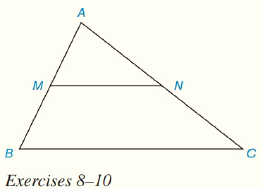Chapter 4.CT, Problem 10CTElementary Geometry For College St...

7th Edition
Alexander + 2 others
ISBN: 9781337614085

Solutions

Chapter
SectionElementary Geometry For College St...

7th Edition
Alexander + 2 others
ISBN: 9781337614085
Textbook Problem

In Δ A B C , M is the midpoint of A B ¯ and N is the midpoint of A C ¯ . If MN= 3x-11 and BC= 4x+24, find the value of x.To determine

To Find:

Value of x.

Explanation

Given:

M is the midpoint of AB¯ and N is the midpoint of AC¯

Calculation:

The line joining the midpoints of two sides of a triangle is parallel to the third side.

Therefore

MN=12(BC)

Still sussing out bartleby?

Check out a sample textbook solution.

See a sample solution

The Solution to Your Study Problems

Bartleby provides explanations to thousands of textbook problems written by our experts, many with advanced degrees!

Get Started

In Exercises 6372, evaluate the expression. 65. 12+41612

Applied Calculus for the Managerial, Life, and Social Sciences: A Brief Approach

If f(x)=0xx2sin(t2)dt, find f(x).

Single Variable Calculus: Early Transcendentals, Volume I

Find f. f(x) = 2x + 3ex

Calculus: Early Transcendentals

Find the exact length of the curve. 20. y = 1 ex, 0 x 2

Single Variable Calculus: Early Transcendentals

Given: ABC with BDCE m3=m4=30m1=m2=70 Find: mB

Elementary Geometry for College Students

True or False: is monotonic.

Study Guide for Stewart's Single Variable Calculus: Early Transcendentals, 8th

Find the present value of 38000 due in 3 years at 814%/year compounded quarterly.

Finite Mathematics for the Managerial, Life, and Social Sciences

j × (−k) = i −i j + k −j − k

Study Guide for Stewart's Multivariable Calculus, 8th